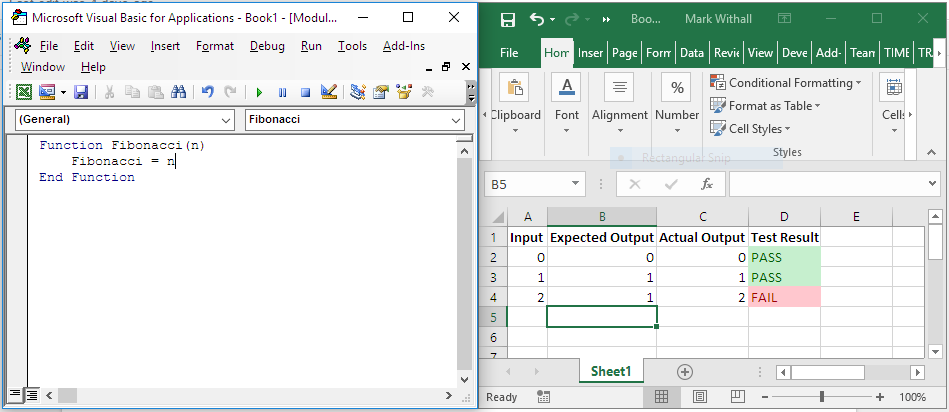Posted by Mark Withall: 2016-09-16

Every so often, I have contemplated the idea of TDD for Microsoft Excel VBA. Finally, I got around to trying it out.

## The Fibonacci Sequence Kata

As a test case, I’m using the Fibonacci Sequence kata by Jason Gorman of Codemanship; as it’s one of the simplest katas out there.

Write a [VBA] script that writes out the number for a given position in the Fibonacci Sequence.

## Step-By-Step

To getting going with TDD in Microsoft Excel VBA we take the following steps:

• Launch Excel
• Create new blank workbook
• Open the Macro Editor (Alt+F11)
• Right-click on current workbook in Macro Editor and insert new module

Now we have everything we need we can write our first failing test. We enter the following in the worksheet:

Input Expected Output Actual Output Test Result
`0` `0` `=Fibonacci(A2)` `=IF(B2=C2,"PASS","FAIL")`

Conditional formatting can be added to the Test Result column to better highlight PASS and FAIL.

Now we have our first failing test, we can add code to make the test pass in the macro editor:

``````Function Fibonacci(n)
Fibonacci = 0
End Function
``````

Switching back to the worksheet and pressing F9 refreshes the test results. We can now see the first test pass.

We now add the next test:

Input Expected Output Actual Output Test Result
`0` `0` `=Fibonacci(A2)` `=IF(B2=C2,"PASS","FAIL")`
`1` `1` `=Fibonacci(A3)` `=IF(B3=C3,"PASS","FAIL")`

And update code to make it pass:

``````Function Fibonacci(n)
Fibonacci = n
End Function
``````

Repeat until no more failing tests can be added.Input Expected Output Actual Output Test Result
`0` `0` `=Fibonacci(A2)` `=IF(B2=C2,"PASS","FAIL")`
`1` `1` `=Fibonacci(A3)` `=IF(B3=C3,"PASS","FAIL")`
`2` `1` `=Fibonacci(A4)` `=IF(B4=C4,"PASS","FAIL")`
``````Function Fibonacci(n)
If n < 2 Then
Fibonacci = n
Else
Fibonacci = 1
End If
End Function
``````
Input Expected Output Actual Output Test Result
`0` `0` `=Fibonacci(A2)` `=IF(B2=C2,"PASS","FAIL")`
`1` `1` `=Fibonacci(A3)` `=IF(B3=C3,"PASS","FAIL")`
`2` `1` `=Fibonacci(A4)` `=IF(B4=C4,"PASS","FAIL")`
`3` `2` `=Fibonacci(A5)` `=IF(B5=C5,"PASS","FAIL")`
``````Function Fibonacci(n)
If n < 2 Then
Fibonacci = n
Else
Fibonacci = n - 1
End If
End Function
``````

Skipping 4, as it already passes:

Input Expected Output Actual Output Test Result
`0` `0` `=Fibonacci(A2)` `=IF(B2=C2,"PASS","FAIL")`
`1` `1` `=Fibonacci(A3)` `=IF(B3=C3,"PASS","FAIL")`
`2` `1` `=Fibonacci(A4)` `=IF(B4=C4,"PASS","FAIL")`
`3` `2` `=Fibonacci(A5)` `=IF(B5=C5,"PASS","FAIL")`
`5` `5` `=Fibonacci(A6)` `=IF(B6=C6,"PASS","FAIL")`
``````Function Fibonacci(n)
If n < 2 Then
Fibonacci = n
Else
Fibonacci = Fibonacci(n - 1) + Fibonacci(n - 2)
End If
End Function
``````That completes the “Happy Path”. One can continue with negative numbers and other invalid input.

## Conclusions

We’ve shown that it is possible to do TDD in Microsoft Excel VBA (at least for simple functions that return a value).

More complex tests might require something more from our test setup. Perhaps writing test functions in VBA that return “PASS” or “FAIL” (and details) and calling them from cells in the worksheet.